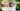# Check whether number is palindrome or not - Leet Code Solution

August 28, 2019## Problem Statement

Determine whether an integer is a palindrome. An integer is a palindrome when it reads the same backward as forward.

``````Example 1:

Input: 121
Output: true

Example 2:

Input: -121
Output: false
Explanation: From left to right, it reads -121. From right to left, it becomes 121-. Therefore it is not a palindrome.

Example 3:

Input: 10
Output: false``````

## Algorithms

There can be many solutions to this:

1. Convert number to character array, and keep on matching characters.
2. Reverse whole number and compare
3. Get the length of number, and iterate over number digit by digit

## Solution - Reverse number and compare

``````public class Q9_Palindrome {
private int reverseInt(int x) {
int s = 0;
while (x > 0) {
s = s*10 + x%10;
x = x/10;
}
return s;
}

public boolean isPalindrome(int x) {
if (x < 0) return false;
return x == this.reverseInt(x);
}
}``````

## Solution - Iterate over number digit by digit

``````public class Q9_Palindrome {
/**
* Example: 1234
* place=0, result=4
* place=1, result=3
*/
private int getDigit(int x, int place) {
x = x / (int)Math.pow(10, place);
return x % 10;
}

public boolean isPalindrome(int x) {
if (x < 0) return false;
int l = 0;
int temp = x;
while (temp > 0) {
l++;
temp /= 10;
}

for (int i=0; i<l; i++) {
if (this.getDigit(x, i) != this.getDigit(x, l-1-i)) {
return false;
}
}
return true;
}
}``````